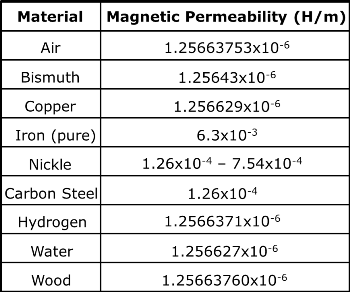# What is meant by “changing magnetic field”

At first I thought it was the consequence of an object moving from an area of high to low or low to high flux density, but that doesn’t help understand what is meant by “a changing magnetic field” inducing a voltage on a transformers secondary coil. So what is a changing magnetic field?Now that I understand that you're referring to transformers, and that you're a GCSE student, I think I have a more appropriate answer to your question, in a way I would have understood when I did my GCSE.

In the case of a transformer the changing magnetic field is due to the fact that the current in the primary coil is an alternating current. In AC systems both the current and the voltage change over time. Indeed they have a sinusoidal wave pattern. If you haven't already done so in GCSE, you will derive this in A-Level physics and see exactly why this is the case, but it's everything to do with how electricity is generated (by a spinning magnet). Below I've linked a plot of DC current (top) and voltage against AC (bottom).

DC current and voltage and AC current and voltage.

I take it that you have learned that charge carrying wires produce magnetic fields. The alternating current in the primary wire then induces a magnetic field in the iron that also alternates, i.e. changes over time, like the current. It is this changing magnetic field that then induces a current in the secondary coil, this time with a lower voltage.

It's helpful to remember that the primary and secondary windings of a transformer are essentially inductors in a circuit that are wrapped around and iron core for a strength boost. With that in mind, it's helpful to talk about inductors.

$$\textit{Inductors:}$$

The entire purpose of an inductor in a circuit is to store energy in a magnetic field. As current runs through the inductor, the $$\vec B$$ field lines develop as in the diagram below.$$\textit{DC/AC:}$$

Now in a DC circuit, after enough time, the field builds up in opposition to current, but over enough time, the magnetic field reaches its maximum value and the circuit continues normally. In this case, you have a static magnetic field. However, in an AC circuit, the voltage and consequently the current are constantly changing. This means that the magnetic field is building up and collapsing constantly in the circuit. In other words, it is producing a changing magnetic field over time. Remember, according to Faraday's Law, it is only a changing magnetic field that will induce voltage elsewhere. Now that we know the one of our transformer sides is producing this changing magnetic field, lets look back at the whole situation.$$\textit{Two Inductors (A Transformer):}$$

You can see that the magnetic field from the primary is passing through the secondary coil. Since this is an AC circuit and our current changes, our magnetic field also changes. This causes a potential difference (Voltage) to be produced in the secondary windings due to induction.

$$\textit{Iron Core:}$$

Now we can stick the iron core back inside the windings and use it to amplify the magnetic field since iron is superior to air in magnetic permeability.$$\textit{Summary:}$$

What is meant by a changing magnetic field is that over time in a transformer, an AC circuit is storing up and breaking down its magnetic field in opposition to the change in current of a circuit.